# How to efficiently implement k Queues in a single array?

## Introduction

Almost all of us have waited in long queues at the ticket counter and bus stops. The idea is simple: whoever will come first will get the ticket first. The person who is coming last is getting the tickets last.In computer programming, the Queue data structure is similar to the real-life queues. The Queue data structure is an ordered collection of items wherein the addition of new items happens at one end called the rear, and the deletion of new items happens at the other end called the front. This ordering principle is called First-In-First-Out or FIFO.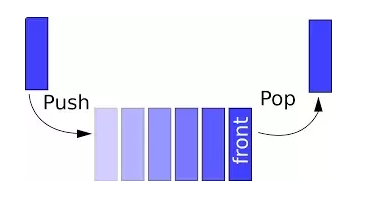Queues are an important data structure from an interview perspective. Almost all the companies ask questions related to queues in the initial stages. This blog will discuss one such important question: Implementation of K queues in a single array.

## Implement K Queues in a single array: Problem Statement

The problem statement says, "You need to implement a data structure KQueues that represents K queues in a single array." The data structure KQueues should support the following operations.”

1. enqueue(int x, int Qn): Inserts an element x to queue number Qn where Qn ranges from 0 to K-1
2. dequeue(int Qn): Deletes an element from queue number Qn where Qn ranges from 0 to K-1.

The user will input the number of queues they wish to implement and the number of elements in each queue.

It is crucial to understand that if there are K queues in a single array, then each queue should satisfy the following conditions:

• Insertion of new elements will always happen from the rear end of the queue.
• Deletion of an element will always happen from the front of the queue.
• While inserting and deleting elements in a queue, a continuous check for overflow and underflow needs to be done.

Another important point to keep in mind while designing a data structure for implementing K queues in a single array is that the queue is open at both ends. One end of the queue is open for insertion, and the other end of the queue is open for deletion operation.

## Approach 1: Implement K Queues in a Single Array

Once the user has input the number of queues and the number of elements in each queue, the total number of elements can be calculated as:

Total number of elements(N) = Number of Queues(k) * Number of Elements in each Queue(n)

It is always recommended to start with the simplest approach, the brute force approach, in an interview. A brute force solution for this problem is creating an array of size N and dividing it into k slots wherein each slot contains N/k elements.

Use the array slots as follows:

• arr to arr[N/k-1] for the first queue.
• arr[N/k] to arr[2N/k-1] for the second queue.
• ………………………………………………….
• ………………………………………………….

For example, suppose if a user wishes to implement 4 queues each containing 3 elements the array, arr[], will look like: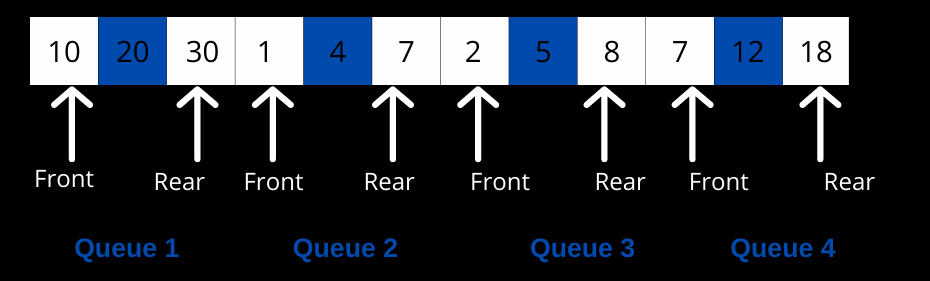This approach is good to think of as a starting point in an interview. While the approach seems efficient in terms of time complexity and is well suited for the requirements. But the problem with this approach is the Inefficient use of array space.

Consider an example wherein we implement four queues, each of size 3 in an array as per the approach discussed above.

The total size of the array will be 4*3 = 12. The CPU will do memory allocation for 12 elements for this array.

Suppose the user enqueue/insert three elements in the first queue and nothing in the second queue, as shown below. Also there are elements in the third and fourth queue as well.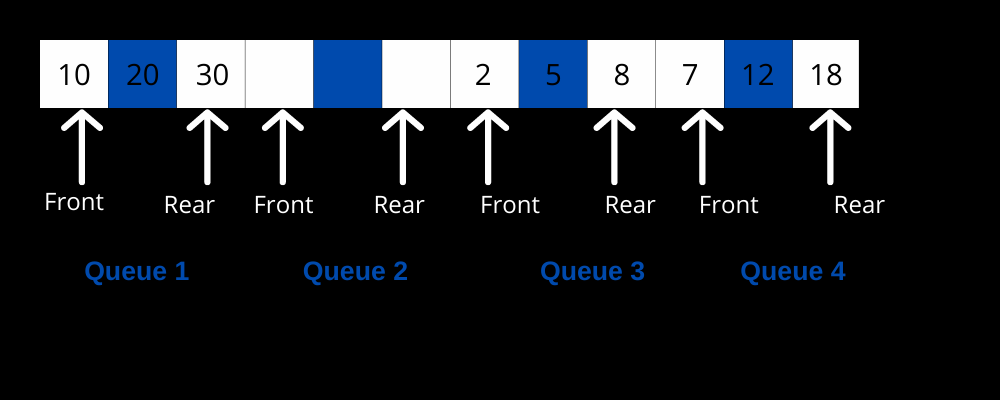Now suppose the user wishes to insert one more element in the first queue. This is possible as far as the array is concerned, however because of the clear division of an array into queues of size three each, insertion of the 4th element will result in Memory overflow, even though there is a space for three more elements in the array.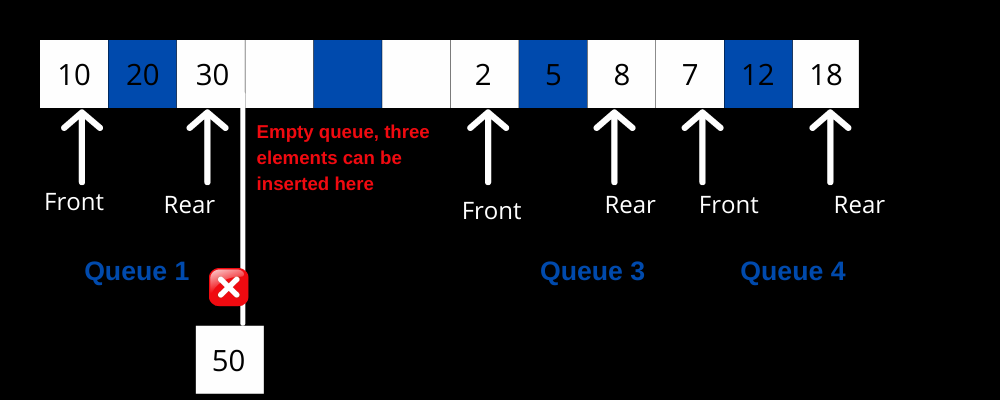The inefficient memory usage may seem irrelevant when dealing with a smaller set of data. However, when working on real-life problems, wherein each queue may contain thousands of elements, and each record is of hundred of bytes, such memory wastage will be too costly and may even cause the program to stop working because of memory overflow.

## Approach 2: Implement K Queues in a Single Array:

The idea is to use three extra arrays to efficiently implement K queues in a single array. Following are the arrays required:

1. frontQueue[]: This array will store the indexes of the front element of the queue. Since there are k queues, the size of this array will be k.
2. rearQueue[]: This array will store the indexes of the rear elements of the queue. Since there are k queues, the size of this array will be k.
3. nextQueue[]: This array will store the indexes of the next item for all items in the array arr[]. Since the number of elements in the array is N, the size of this array will be N.

The frontQueue and the rearQueue array will be initialized with -1 to indicate that all the queues are empty.

The nextQueue[i] will be initialized with i+1 because all the slots are initially free and point to the next slot.

For example, consider that you want to implement four queues each of size 2. The structure of frontQueue, rearQueue and the nextQueue arrays will be as shown below: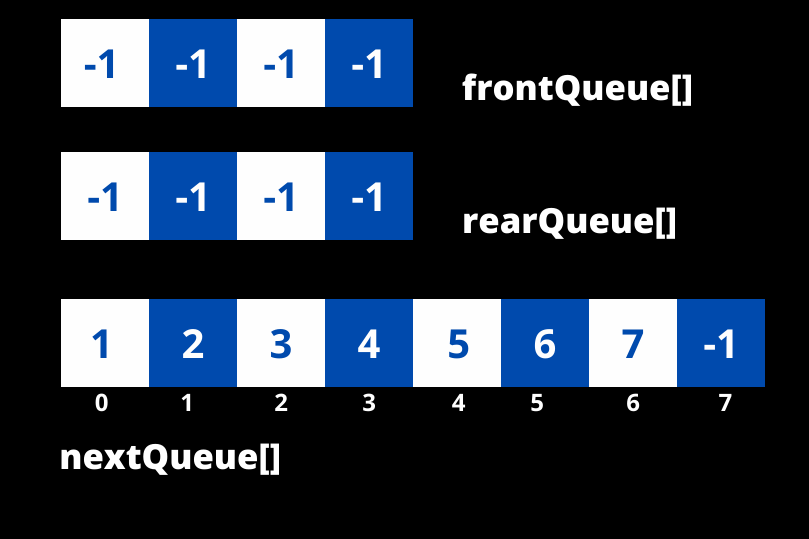Apart from the three auxiliary arrays, an additional stack of free slots in the array called a free stack will be maintained. The top of the free stack will be initialized as 0.

## Understanding Enqueue Operation

Before moving on to the code, let's look at the various factors that need to be encountered during insertion in ith queue:

• The frontQueue[i] and the rearQueue[i] need to be updated.
• frontQueue[i] will be updated when the first element of the ith queue is inserted into the array.
• The rearQueue[i] will be updated for every insertion.
• If the ith queue was initially empty, both the rearQueue[i] and the frontQueue[i] will be set to the top of the free stack.
• If the ith queue was initially not empty, then the rearQueue[i], as well as the nextQueue[rearQueue[i]], will be set to the top of the free stack.

• The nextQueue[i] holds the index value of the next item for the item in array arr[]. So for every valid insertion,  nextQueue[i] will be set to -1, indicating that this is the rearmost element of the ith queue.
• The top of the free stack will be set to the index of the next slot.

## Understanding Dequeue Operation

Dequeue operation always works from the front of the queue. Before moving on to the code, let us look at the various factors that need to be considered while deletion in a queue:

• Always Check for the underflow condition first.
• frontQueue[i] needs to be updated as the deletion happens from the front.
• Store the index(frontIndex) of the front item in the ith queue.
• Change the frontQueue[i] to the nextQueue[frontIndex].
• Set the nextQueue[frontIndex] to the top of the free stack.
• Set the top of the free stack to the frontIndex.

## Implementation

Upon deletion of an element from the queue, the deleted element will be replaced by 0 in the below program.

public class KQueues

{

int k; // denoting the number of queues

int n; // denoting the number of elements in each queue

int[] arr; // will store the queue elements

int[] frontQueue; // will store the front elements of all the k queues

int[] rearQueue; // will store the rear elements of all the k queues

int[] nextQueue; // will store the next elements index

int free;

// Constructor to initialize the values wherein K queues are

// to be implemented in array, each queue is of size n

KQueues(int k, int n)

{

this.k = k;

this.n = n;

this.arr = new int[n];

// The frontQueue and rearQueue will be of size k

this.frontQueue = new int[k];

this.rearQueue = new int[k];

// The nextQueue will be of size n

this.nextQueue = new int[n];

// Initializing the frontQueue and the rearQueue with -1

// indicating all the queues are initially empty

for(int i = 0; i < k; i++)

{

frontQueue[i] = rearQueue[i] = -1;

}

// Initializing all the spaces as free

free = 0;

for(int i = 0; i < n - 1; i++)

{

nextQueue[i] = i+1;

}

//The last element in the nextQueue array will be -1

nextQueue[n-1] = -1;

}  // Constructor ends here

// Method to check if queue number i is empty.

// This method will return true if frontQueue[i] is -1 indicating

// empty ith queue

private boolean isQueueEmpty(int i)

{

return frontQueue[i] == -1;

}

// Methd to check of queue number i is full

// This method will return true if free is -1

private boolean isQueueFull(int i)

{

return free == -1;

}

// Method to insert an item in queue number q

private void enqueue(int item, int q)

{

// Firstly check if the queue, 'q' is completely full.

if(isQueueFull(q))

{

System.out.println("Queue Overflow");

return;

}

// New element will be inserted in the next free space available

// in the array. The nextQueue[] array is used for this purpose.

int nextFreeSpace = nextQueue[free];

// Case where the qth queue was empty then both the frontQueue[q]

// as well as the rearQueue[q] needs to be updated

if(isQueueEmpty(q)) {

rearQueue[q] = frontQueue[q] = free;

// Case where the qth queue is not empty, in this case only the

// rearQueue[] and the nextQueue[] needs to be updated.

}else {

// Update next of rear and then rear for queue number 'j'

nextQueue[rearQueue[q]] = free;

rearQueue[q] = free;

}

nextQueue[free] = -1;

// Put the item in array

arr[free] = item;

// Update index of free slot to index of next slot in free list

free = nextFreeSpace;

} // Method ends here

// Method for removal of an item from the qth queue.

private int dequeue(int q)

{

// Underflow condition check

if(isQueueEmpty(q)){

System.out.println("STACK UNDERFLOW");

return Integer.MIN_VALUE;

}

// Find the index of the front item in qth queue

int frontIndex = frontQueue[q];

// Changing the value of frontQueue[q]

frontQueue[q] = nextQueue[frontIndex];

nextQueue[frontIndex] = free;

free = frontIndex;

int value = arr[frontIndex];

arr[frontIndex] = 0;

return value;

}

// The print function works as follows:

// The frontQueue[q] stores the index of front element of qth queue of the array, arr[]

// The rearQueue[q] stores the index of rear elements of the qth queue of the array, arr[]

// So in order to print the qth queue, start from the frontQueue[q]th index and print the

// elements till the rearQueue[q]th index.

public void printQueue(int q)

{

int start = frontQueue[q];

int end = rearQueue[q];

while(start <= end)

{

System.out.print(arr[start] + " ");

start++;

}

}

public static void main(String[] args)

{

// Creating 3 queues each of size 3

int k = 4, n = 20;

KQueues obj = new KQueues(k, n);

// Inserting 10 in the first queue

obj.enqueue(10, 1);

// Inserting 20 in the first queue

obj.enqueue(20, 1);

// queue 1 is now 10->20

// Inserting 1 in the second queue

obj.enqueue(1, 2);

// Inserting 4 in the second queue

obj.enqueue(4, 2);

// Inserting 9 in the second queue

obj.enqueue(9, 2);

// Inserting 19 in the second queue

obj.enqueue(19, 2);

// queue 2 is now 1->4->9->19

// Inserting 7 in the third queue

obj.enqueue(7, 3);

// Inserting 5 in the third queue

obj.enqueue(5, 3);

// Inserting 8 in the third queue

obj.enqueue(8, 3);

// queue 3 is now 7->5->8

System.out.println("Printing the first queue");

obj.printQueue(1);

System.out.println("\nDequeued element from queue 1: " + obj.dequeue(1));

System.out.println("Printing the first queue after dequeue operation");

obj.printQueue(1);

System.out.println("\nPrinting the second queue");

obj.printQueue(2);

System.out.println("\nDequeued element from queue 2: " + obj.dequeue(2));

System.out.println("Printing the second queue after dequeue operation");

obj.printQueue(2);

System.out.println("\nPrinting the third queue");

obj.printQueue(3);

System.out.println("\nDequeued element from queue 3: " + obj.dequeue(3));

System.out.println("Printing the third queue after dequeue operation");

obj.printQueue(3);

}

}

The output of the above program is:

The time and space complexity of the enqueue() and dequeue() operation is O(1).

The best part of the above implementation is that if there is a slot/empty space available in the queue, an item can be enqueued in any of the queues.

Q1) What is the difference between Stacks and Queues?
Ans 1) A stack is a linear data structure in which elements can be inserted and deleted only from one end. Stack follows the Last In First Out(LIFO) principle.

While a queue is based on the First In First Out principle, the insertion of elements occurs at the rear end, while the deletion takes place at the front end.

Q2) Can we implement a queue using Stacks?
Ans 2) A queue can be implemented using Stacks. Refer to the blog for more information.

Q3) How to implement K queues in a single array?
Ans 3) To implement K queues in a single array, the following approaches can be used:

## Key Takeaways

The article discussed an interview problem: Implement K queues in a single array. With this done, you may now practice questions related to Stacks and Queues on Codestudio. Remember, the more you practice, the better your problem-solving skills are. Codestudio has the best-structured content for interview problems as well, do give it a try.

While Queues are an important topic from an interview perspective, there are other important topics as well. You must check out Codestudio for free guided paths on Data Structure and AlgorithmsSystem Design, and Competitive Programming.

Remember Practice is the Key!!